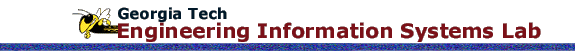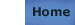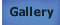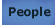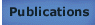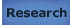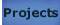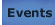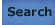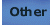# Knowledge Composition for Efficient Analysis Problem Formulation Part 1: Motivation and Requirements

## Citation

Bajaj M, Peak RS, Paredis CJJ (2007) Knowledge Composition for Efficient Analysis Problem Formulation - Part 1: Motivation and Requirements. Paper DETC2007-35049, Proc ASME CIE Intl Conf, Las Vegas.

## Keywords

simulation-based design (SBD), problem formulation, CAD-CAE interoperability, design-analysis integration (DAI), knowledge composition methodology (KCM), variable topology multi-body (VTMB) problem, model transformation, NIST Core Product Model (CPM2), SysML

## Abstract

In simulation-based design a key challenge is to formulate and solve analysis problems efficiently to evaluate a variety of design alternatives. Numerically solving analysis problems has benefited from advancements in commercial off-the-shelf mathematical solvers and computational capabilities. However, the formulation of analysis problems for a given set of design alternatives is still typically a laborious and costly process. In the scope of design alternatives with variable topology multi-body (VTMB) characteristics, these papers (Part 1 and Part 2) present research that addresses the following primary question:

How can we improve the efficiency of the analysis problem formulation process for VTMB design alternatives?

The objective of this paper (Part 1) is to identify requirements for a methodology that answers this. The methodology is formulates analysis problems for VTMB design alternatives based on decisions taken by analysts and independent of the solution method (such as finite element analysis) and the solver. This paper presents a gap analysis using an example VTMB problem and identifies key inadequacies in existing approaches for analysis problem formulation. Based on the gap analysis and technical background, we present five main requirements relating to (a) key drivers for efficiently creating analysis models; (b) abstracting and formalizing analysis knowledge for composing analysis models; and (c) automatically creating, reconfiguring and verifying analysis models.